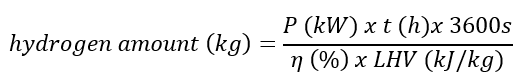# How much hydrogen do I need for the fuel cell on my ship?

• Home• How much hydrogen do I need for the fuel cell on my ship?

## HOW MUCH HYDROGEN DO I NEED FOR THE FUEL CELL ON MY SHIP?

How much hydrogen is needed to run a x number of hours with a fuel cell of a certain capacity on your ship? We've got the answer.

The amount of hydrogen required depends on the efficiency of the fuel cell. This is higher when the fuel cell is new compared to the end of its life. Original equipment manufacturers (OEM's) use the term beginning of life (BOL) and end of life (EOL). The efficiency of a fuel cell BOL is approximately 51%, EOL is approximately 45%

In addition to efficiency, we need the energetic value or more specific the lower heating value (LHV) of hydrogen to determine the hydrogen consumption, which is 120,000 kJ / kg.

With these values ​​we can easily calculate how much hydrogen is needed using the formula below:For example: To run a 200 kW fuel cell for 8 hours, (200 kW x 8 hours x 3600s) / (51% x 120,000 kJ / kg) = 94 kg of hydrogen is required.

A type 4 hydrogen cylinder under a pressure of 300 bar (30 MPa) contains approximately 7 kg of hydrogen. In the example above, 13 pieces of cylinders are therefore required. The cylinders have a diameter of 515mm and are approximately 2.6m long.

 Fuel cell power (kW) Operating time (h) Fuel cell efficiency (%) Required amount of hydrogen (kg)
Want to know more about our hydrogen solutions?